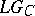# Loop group

There are different spaces known as "loop groups" . One of the most studied is defined by considering, for $G$ a compact semi-simple Lie group (cf. also Lie group, semi-simple), the loop group $\Omega G = \{ \gamma : S ^ { 1 } \rightarrow G : \gamma ( 1 ) = 1 \}$; here, $S ^ { 1 }$ is the circle of complex numbers $z = e ^ { i \theta }$.

One can take spaces of polynomial, rational, real-analytic, smooth, or $L _ { 1 / 2 } ^ { 2 }$ loops, in decreasing order of regularity. The metric $L _ { 1 / 2 } ^ { 2 }$ is actually a Kähler metric. The complex structure is defined by considering as $( 1,0 )$-forms on the Lie algebra of $\Omega G$, those with positive Fourier coefficients. Equivalently, $\Omega G$ is a homogeneous space of the loop group $L G _ { \text{C} } = \{ \gamma : S ^ { 1 } \rightarrow G _ { \text{C} } \}$, with isotropy

\begin{equation*} L^+G _ { \mathbf{C} } = \left\{ \begin{array}{l}{ \\ \gamma \in L G _ { \mathbf{C} } :\\ }\end{array} \begin{array}{c}{ \gamma \text{ extends} \\ \text{ holomorphically in the disc } }\\{ \text { to a group } "\square" \text{valued mapping }}\end{array} \right\}. \end{equation*}

This is shown by considering the "Grassmannian model" of D. Quillen for $\Omega G$. For simplicity, take $G = U ( n )$; then $\Omega U ( n )$ is naturally identified with the Hilbert–Schmidt Grassmannian of certain Hilbert subspaces of $L ^ { 2 } ( S ^ { 1 } , \mathbf{C} ^ { n } )$, stable under multiplication by $z$. This also shows that $\Omega G$ has a holomorphic embedding into a projectivized Hilbert space, by generalized Plücker coordinates; and a determinant line bundle.

While the smooth loop group is an infinite-dimensional Fréchet manifold, the rational and polynomial loop groups have natural filtrations by finite-dimensional algebraic subvarieties. Moreover, by a result of G. Segal, any holomorphic mapping from a compact manifold into $\Omega G$ actually goes into the rational loops (up to multiplication by a constant). This has application in gauge theory, because a theorem of M.F. Atiyah and S.K. Donaldson identifies, for a classical group $G$, the moduli space of charge-$k$ framed $G$-instantons with the moduli spaces of based holomorphic $2$-spheres in $\Omega G$, of topological degree $k$. Another application to gauge theory and to the theory of completely integrable systems is given by a construction of K. Uhlenbeck, refining earlier work by V.E. Zakharov and others: this identifies, modulo basepoints, harmonic mappings $f : S ^ { 2 } \rightarrow G$ with certain holomorphic mappings $F : S ^ { 2 } \rightarrow \Omega G$. The degree $k$ of $F$ now gives the energy of $f$, by a formula of G. Valli, generalizing earlier work of E. Calabi.

The representation theory ofhas also been studied: the key point is the construction of a universal central extension, which makes it possible to define infinite-dimensional projective representations.

Other spaces commonly known as "loop groups" are the group of diffeomorphism of the circle, $\operatorname{Diff}( S ^ { 1 } )$, and the loop space of a manifold, $LM$.

The space $\operatorname{Diff}( S ^ { 1 } )$ has been studied as reparametrization groups for string theory. It has two natural homogeneous spaces: $\operatorname{Diff} ( S ^ { 1 } ) / \operatorname{SL} ( 2 , {\bf R} )$ and $\operatorname{Diff}( S ^ { 1 } ) / S ^ { 1 }$, which are infinite-dimensional Kähler manifolds (the Kähler metric is the metric $L _ { 3 / 2 } ^ { 2 }$). The space $\operatorname{Diff} ( S ^ { 1 } ) / \operatorname{SL} ( 2 , {\bf R} )$ has been studied in the theory of universal Teichmüller spaces (cf. also Teichmüller space).

When $M$ is a manifold, $LM$ is defined as the space of loops in $M$ starting at a fixed basepoint. Here, the group operation is given by composition of loops. The space $LM$ has been considered in connection with the problem of characterizing parallel transport operators, as configuration space in string theory, and in probability theory.

How to Cite This Entry:
Loop group. Encyclopedia of Mathematics. URL: http://encyclopediaofmath.org/index.php?title=Loop_group&oldid=53840
This article was adapted from an original article by Giorgio Valli (originator), which appeared in Encyclopedia of Mathematics - ISBN 1402006098. See original article